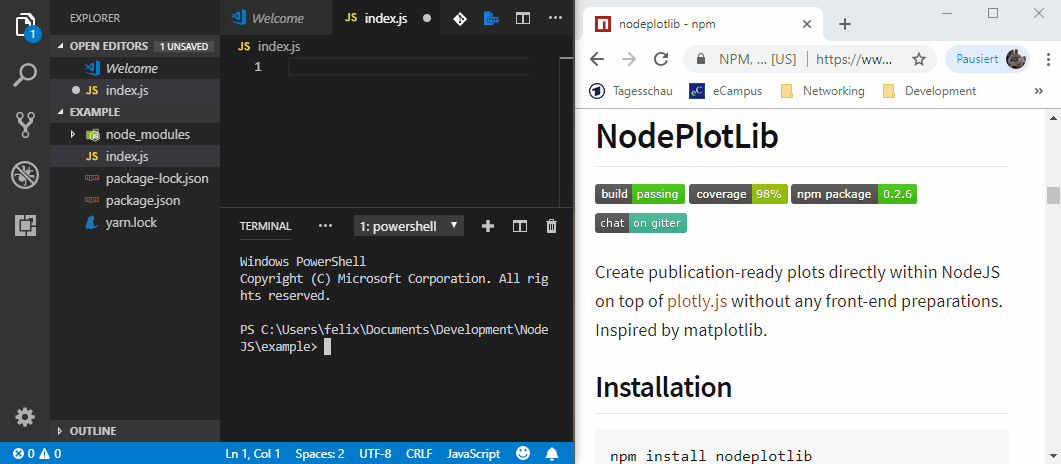Nutrient Packed Morsels

# npm

Wondering what’s next for npm?Check out our public roadmap! »

## nodeplotlib0.7.3 • Public • Published

# NodePlotLib

Library to create top-notch plots directly within NodeJS on top of plotly.js without any front-end preparations. Inspired by matplotlib.## Installation

npm install nodeplotlib
# or
yarn add nodeplotlib

## Usage

### Overview

Use with TypeScript/JavaScript:

import { plot, Plot } from 'nodeplotlib';
const data: Plot[] = [{x: [1, 3, 4, 5], y: [3, 12, 1, 4], type: 'line'}];
plot(data);

If ES5 use require() instead of import. Here is a short animation about howto and the results.

### Detailed usage

Since Python provides with matplotlib a library for spawning plot windows, NodeJS isn't by default. But there are awesome plotting libraries for usage in front-end. So this lib targets people like scientists who easily want to create beautiful plots in a time-saving way.

The library provides a simple interface with (for now) just three functions. A plot, stack and a clear function. The plot() functions spawns a plot to the browser, if a plotdata is given as an argument. Otherwise it plots all the stack()ed plotdata. The arguments are of type Plot, which is a partial of plotly's PlotData type. With the clear() function the stack container can be cleared.

With the stack function the user is able to print multiple charts on one page.

import { plot, stack, clear, Plot } from 'nodeplotlib';

const data: Plot[] = [{
x: [ 1, 3, 4, 6, 7],
y: [ 2, 4, 6, 8, 9],
type: 'scatter'
}];

stack(data);
stack(data);
stack(data);
plot();

The plot function plots all stacked plots and the plot given by parameter (if there is one). Afterwards the temporary container gets cleared and you can call stack() and plot() again without any predefined plots.

The functions are of the form:

import { plot, stack, clear, Plot, Layout } from 'nodeplotlib';

plot(data?: Plot[], layout?: Layout): void;
stack(data: Plot[], layout?: Layout): void;
clear(): void;

## Quick start

In this section there are some examples to getting started. See the full plotly cheatsheet.

#### Line Plots

const trace1: Plot = {x: [1, 2], y: [1, 2], type: 'scatter'};
const trace2: Plot = {x: [3, 4], y: [9, 16], type: 'scatter'};
plot([trace1, trace2]);

#### Bar Charts

const trace: Plot = {x: [1, 2], y: [1, 2], type: 'bar'};
plot([trace]);

#### 3D Line Plots

const trace: Plot = {x: [9, 8, 5, 1], y: [1, 2, 4, 8], z: [11, 8, 15, 3], type: 'scatter3d'};
plot([trace]);

#### 3D Surface Plots

const trace: Plot = {colorscale: 'Viridis', z: [[3, 5, 7, 9], [21, 13, 8, 5]]};
plot([trace]);

#### Radial Plots

In order to style the plot, one is able to pass in the layout parameter, which internally is typeof Partial<Layout> from plotly's Layout. See the full layout documentation here.

With this parameter one is able to define styles like title, axis labels, subplots and many more.

const data: Plot[] = [{
type: 'scatterpolar',
r: [1.5, 10, 39, 31, 15, 1.5],
theta: ['A','B','C', 'D', 'E', 'A'],
fill: 'toself',
name: 'Group B'
}];

const layout: Layout = [
polar: {
radialaxis: {
visible: true,
range: [0, 50]
}
}
];

plot(data, layout);

## Plot types

Simple charts Advanced charts 3D Plots
Scatter 2d density plots Scatter
Line Histograms Surface
Bar Box-plots Lines
Pie charts Contour plots
Sankey diagrams Heatmaps
Tables Radar charts

## Behind the scenes

The lib launches a webserver and opens new tabs for every plot located at http://localhost:8080/plots/:id. At this address a temporary html template file, the nodeplotlib script and plotly.min.js are available. The client side js requests the plot data at http://localhost:8080/data/:id. After all pending plots are opened in a unique tab and all the data is requested, the server shuts down. If you fire another plot the server starts again provides your plot and shuts down automatically.

Another port can be provided via PORT environment variable.

## Contributing

Contributions in all forms are welcome.

## Developers guide

Fork the Github repository and clone it to your PC. Install the npm dependencies using the install command. It installs the dependencies and copies plotly types to project source. These won't affect the git tree.

## Roadmap

It would be nice to make the library compatible with Observable-streams and update the plots in real-time.

## Keywords

### Install

npm i nodeplotlib

243

0.7.3

MIT

19.7 kB

12

### Homepage

github.com/ngfelixl/nodeplotlib#readme

### Repository

github.com/ngfelixl/nodeplotlib